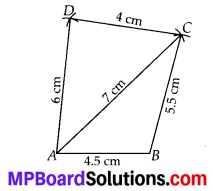# MP Board Class 8th Maths Solutions Chapter 4 Practical Geometry Ex 4.1

## MP Board Class 8th Maths Solutions Chapter 4 Practical Geometry Ex 4.1

Question 1.
ABCD
AB = 4.5 cm
BC= 5.5 cm
MP= 5 cm
AC = 7 cmJUMP
JU = 3.5 cm
UM = 4 cm
MP = 5 cm
PJ = 4.5 cm
PU = 6.5 cm

(iii) Parallelogram
MORE
OR = 6 cm
RE = 4.5 cm
EO = 7.5 cm

(iv) Rhombus
BEST
BE = 4.5 cm
ET = 6 cm
Solution:
(i) Steps of Construction :
Step-1: Draw AB = 4.5 cm.
Step-2: With B as centre and radius equal to 5.5 cm, cut an arc.
Step-3 : With A as centre and radius equal to 7 cm, cut another arc on the arc drawn in step-2 at point C.
Step-4 : With C as centre and radius equal to 4 cm, cut off an arc. Also, with A as centre and. radius equal to 6 cm cut off another arc on the arc just drawn at point D.
Step-5 : Join BC, AC, CD and AD.
Hence, ABCD is the required quadrilateral.(ii) Steps of Construction :
Step-1: Draw JU = 3.5 cm.
Step-2: With J as centre and radius equal to 4.5 cm, cut an arc.
Step-3 : With U as centre and radius equal to 6.5 cm, cut another arc on the arc drawn in step-2 at point P.
Step-4: With P as centre and radius equal to 5 cm, cut off an arc.
Step-5: With U as centre and radius equal to 4 cm, cut another arc on the arc drawn in step-4 at point M.
Step-6 : Join JP, UP, UM and PM. Thus, JUMP is the required quadrilateral.(iii) We know that opposite sides of a parallelogram are equal and parallel to each other.
∴ OR = ME and MO = ER.
Steps of Construction:
Step-1: Draw OR = 6 cm.
Step-2 : With R as centre and radius equal to 4.5 cm, cut an arc.
Step-3 : With O as centre and radius equal to 7.5 cm, cut another arc on the arc drawn in step-2 at point E.
Step-4: With E as centre and radius equal to 6 cm, cut an arc.
Step-5 : With O as centre and radius equal to 4.5 cm, cut an arc on the arc drawn in step-4 at point M.
Step-6 : Join RE, OE, OM and ME.
Hence, MORE is the required parallelogram.(iv) We know that all four sides of a rhombus are equal.
∴ BE = ES = ST = BT = 4.5 cm.
Steps of Construction:
Step-1: Draw BE = 4.5 cm.
Step-2: With B as centre and radius equal to 4.5 cm, draw an arc.
Step-3: With E as centre and radius equal to 6 cm, draw another arc, cutting the previous arc at point T.
Step-4: With E as centre and radius equal to 4.5 cm, cut an arc.
Step-5: With T as centre and radius equal to 4.5 cm, cut another arc on the previous arc at point S.
Step-6 : Join BT, ES, ET and ST.
Hence, BEST is the required rhombus.